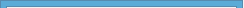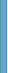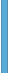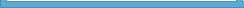About UsContact UsProductsServicesNewsletter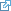Help Forums# Convert Units and Measurements

Convert all kinds of measurements and units with ease in your webbrowser.

## Unit Converter to Convert Units and Measurements Online

• Length unit conversion : metric, millimeter (mm), meter (m), kilometer (km), inch, foot / feet, yard, mile
• Area unit conversion : square (sq), centimeter (cm), meter (m), inch, foot / feet, acre, hectare (ha)
• Volume unit conversion : cubic (cb), centimeter (cm), meter (m), inch, foot / feet
• Weight unit conversion : gram (g), kilogram (kg), grain (gr), ounce (oz), pound (lb), short ton (ston)
• Temperature unit conversion : Celsius, Fahrenheit, Reaumur, Kelvin
• Fluid unit conversion : milliliter (ml), liter (l), ounce (oz), pint (pt), gallon (gal), bushel (bu)

## Unit Conversion Tools in Free Online Unit Converter

Our free unit converter tools can solve a wide variety of unit conversion needs. By using the unit conversion calculator tools you can convert between metric measurements, lengths / distances, areas, weights, volumes, temperatures, and fluids / liquids.

Unlike many unit conversion software programs and online services that require you to click back and forth to each specific unit conversion tool, we group and keep related unit conversion tools together. This makes it easy to quickly to convert units and measurements.

Use our free unit converter tools underneath to create unit conversion tables and learn the factors, scales and math functions behind unit conversion.

 Unit convert length measurement metric, millimeter (mm), meter (m), kilometer (km), inch, foot / feet, yard, mile
 Unit convert area measurement square (sq), centimeter (cm), meter (m), inch, foot / feet, acre, hectare (ha)
 Unit convert volume measurement cubic (cb), centimeter (cm), meter (m), inch, foot / feet
 Unit convert weight measurement gram (g), kilogram (kg), grain (gr), ounce (oz), pound (lb), short ton (ston)
 Unit convert temperature measurement Celsius, Fahrenheit, Reaumur, Kelvin
 Unit convert fluid measurement milliliter (ml), liter (l), ounce (oz), pint (pt), gallon (gal), bushel (bu)© Copyright 1997-2019 Microsys about | contact | feedback | sitemap and search | press | newsletter | articles | forumsUsage of this website constitutes an accept of our legal, privacy policy and cookies information.